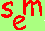David A. Kenny
August 22, 2011

Page recently revised.

Constraints on Parameters:
Phantom Variables

Linear Constraints (parameter a linear function of other parameters)
zero:  a = 0
equality: a = b
negative: a = -b
proportionality: a/b = c/d or a = kb and c = kd
additive: a + b = 1

Non-linear Constraints (less than means less than or equal)
a ≤ 0
a ≤ k
a ≥ k
a ≤ b
a ≤ b + k
a = bc
a = b2

When Constraints Are Needed
Statistical
Force variances to be non-negative (i.e., prevent Heywood cases)
Force correlations to be in range
Test of effects of latent products requires numerous constraints
Repeated measures with Latin squares can require constraints
Theoretical

Proportionality:  For example, if one looks at the effects of mother (M) and father (F) on daughters (D) and sons (S), on might want to force the following constraint:

M → D    F → S

______ = _____

M → S    F → D

That is, a parent has proportionally more influence on a same- than an different-gender child.

Greater than zero:  Over-time stabilities in most models cannot be negative.
Complex constraint:  Certain circumplex models can require that a2 + b2 = k.

Phantom Variables
Variables that have no substantive meaning, but that are created to force constraints on the model. Normally phantom variables have no disturbances. (See D. Rindskopf (1984).  Using phantom and imaginary latent variables to parameterize constraints in linear structural models.  Psychometrika, 49, 37-47.  Some computer programs such as LISREL 8 allow the user to force these constraints without using phantom variables.

How to Use of Phantom Variables to Force Constraints
(The material below is difficult to follow and requires close study.)
The following notation is used:  The effect of X on Y equals a is denoted by X → Y = a.

Phantom variables are denoted as P.

a = -b:  Given X → Z = a and Y → Z = b, the following model is estimated:  Y → Z = b, X → P = -1, P → Z = b.

a/b = c/d or a = kb and c = kd:  Given X → W = a, X → Z = b, Y → W = c, and Y → Z = d, the following model is estimated:  X → Z = b, X → P1 = k, P1 → W = b, Y → Z = d, Y → P2 = k, P2 → W = d.

a + b = k:  Given X → Z = a and Y --> Z = b, the following model is estimated:  X → Z = a, Y → P = -1, P → a, and Y → Z = k.

a ≥ 0:  Given X → Y = a, the following model is estimated:  X → P = b and P → Y = b, making a = b2.

a ≥ k:  Given X → Y = a, the following model is estimated:  X → Y = k and X → P = b and P → Y = b, making a = b2 + k.

a ≥ b:  Given X → Z = a and Y --> Z = b, the following model is estimated:  X → Y = b, Y → Z = b, X → P = c, and P → Z = c, making a = b + c2 and because c2 cannot be negative then a ≥ b.

a ≥ b + k:  Given X → Z = a and Y → Z = b, the following model is estimated:  X → Y = b, Y → Z = b, X → P = c, P → Z = c, and X → Z = k, making a = b + c2 + k.

a = bc:  Given X → W = a, Y → W = b, and Z → W = c, the following model is estimated: Y → W = b, Z → W = c, X → P = a, P → W = b, making a = bc.

a = b2:  Given X → Z = a and Y → Z = b, the following model is estimated:  Y → Z = b, X → P = b, P → Z = b, making a = b2.

Go to the next page.